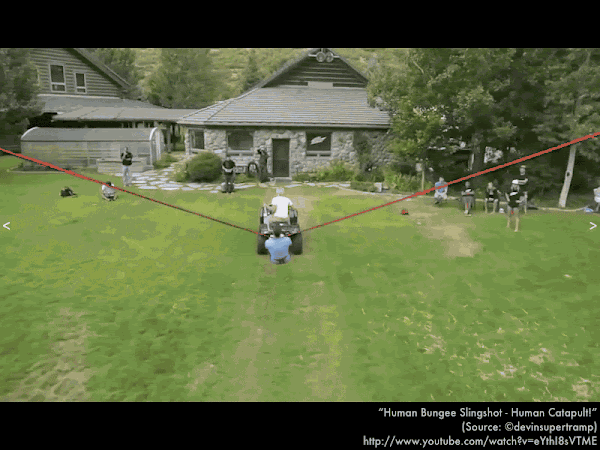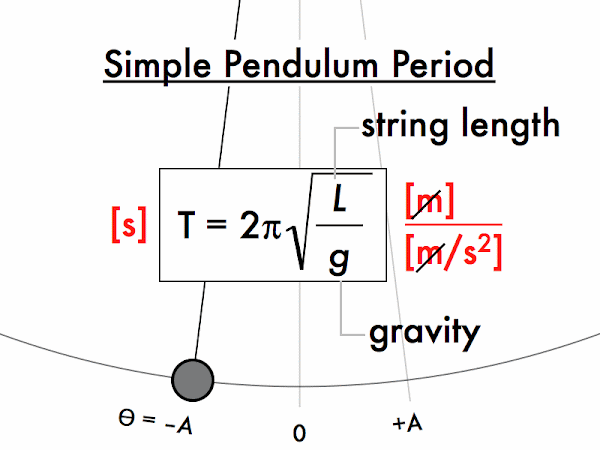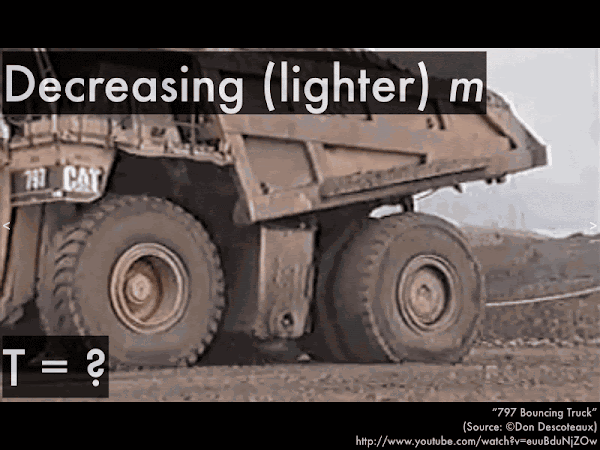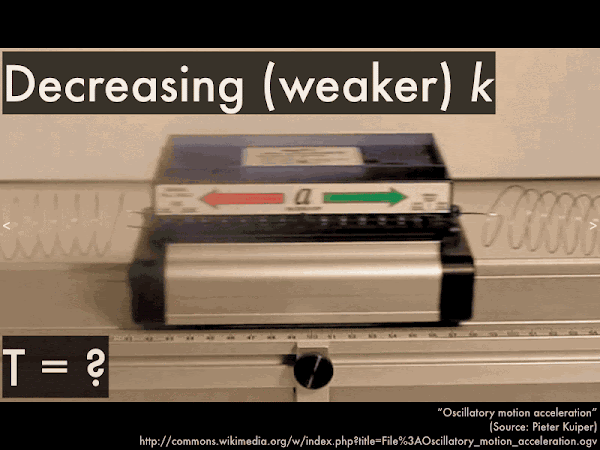## 20141107

### Physics presentation: simple harmonic motionNothing like communing with nature using a harness, an ATV and a set of bungee cords. Did I say communing with nature? I meant to say communing with physics. (Video link: "Human Bungee Slingshot - Human Catapult!.")Or if being slung back-and-forth isn't your thing, you can always swing back-and-forth. Good times. (Video link: "Arch Swing Corona to Tool.")Both the bungee cord sling and the natural arch swing are examples of simple harmonic motion, which we'll analyzing in terms of energy conservation and their periodic behavior.The simple in simple harmonic motion denotes that we will ignore any outside work being done by external sources and dissipative forces such as friction or drag, such that under this ideal condition the back-and-forth motion of the object will continue forever. The harmonic in simple harmonic motion follows from how the position versus time will trace out a sine or cosine function (which has the mathematical property of being proportional to its second derivative). (Video link: "Tom Altman and SHM at the Exploratorium.")First, analyzing simple harmonic motion with energy conservation.Since there are no energy losses (or gains) from the outside world, a mass on a spring undergoing simple harmonic motion will have a constant total mechanical energy E, which is the sum of the translational kinematic energy and elastic potential energy. Even though the relative amounts of KEtr and PEelas may be different, their total must always equal the same constant amount.Let's consider when the mass on this spring would momentarily have no translational kinetic energy, such that all of its total energy was in the form of elastic potential energy. What location(s) would E = PEelas only?Let's consider when the mass on this spring would momentarily have no elastic potential energy, such that all of its total energy was in the form of translational kinetic energy. What location(s) would E = KEtr only?Second, timing the cycles of simple harmonic motion.For a simple pendulum, which is a mass on a string, the period (time for the mass to complete one cycle of motion) depends on the square root of the string length L and the gravitational acceleration g.For these identical mass billiard balls hanging from strings of different lengths, do the billiard balls hanging from shorter length strings have shorter, longer, or the same periods compared to the billiard balls hanging from longer length strings? (Video link: "Pendulum Waves with Philip Glass.")

For two different simple pendulum systems, their periods are given by:

Tshort = (2·π)·√(Lshort/g),

Tlong = (2·π)·√(Llong/g),

and since the factors of 2, π, and g are the same for all these billiard balls, then we can see that the shorter length string will have a shorter period (and will take less time to swing back and forth), while the longer length string will have a longer period (and will take more time to swing back and forth).For the different mass riders on swings of the same lengths, does the more massive rider have a shorter, longer, or the same period compared to the less massive rider? (Video link: "BALOICA.")For a mass on a spring, the period (time for the mass to complete one cycle of motion) depends on the square root of the mass m and the spring constant k.

It is not just the square root and the factor of 2·π in the mass-spring period that is similar to the simple pendulum period--both expressions for periods have an inertial parameter in the numerator under the square root: the length L or the mass m. Both parameters are a measure of how difficult it is to change the rotational or translational motion of the simple harmonic motion object, and increasing the simple pendulum length or the mass attached to a spring would increase the time to complete one cycle of motion.

Likewise, both expressions for periods have a restoring parameter in the denominator under the square root: the gravitational acceleration constant g or the spring constant k. Both are a measure of the torques or the forces exerted on a simple harmonic motion object to return it back towards equilibrium, and increasing the gravitational constant (by changing its location?) or the strength of the spring would decrease the time to complete one cycle of motion.For the overloaded truck with a vertical oscillation period of approximately half a second, what would happen to the period of its motion after dumping its load, thus decreasing the mass connected to the springs in its suspension? Would the period decrease, increase, or remain the same? (Video link: "797 Bouncing Truck.")

For a loaded and an unloaded truck, their periods are given by: